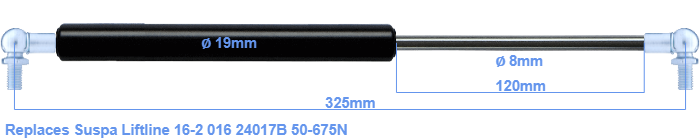# Replacement for Suspa Liftline 16-2 016 24017B 50-675N

\$36.51

Replacement gas spring for the Suspa Liftline 16-2 016 24017B 50-675 Newton. This fits a (possibly already present) ball with a diameter of 10mm. A corresponding ball is included. Brand: Stabilus Industry Line.
 Force Choose an option50 Newton60 Newton80 Newton100 Newton120 Newton140 Newton150 Newton160 Newton180 Newton200 Newton220 Newton240 Newton250 Newton260 Newton280 Newton300 Newton320 Newton340 Newton350 Newton360 Newton380 Newton400 Newton420 Newton440 Newton450 Newton460 Newton480 Newton500 Newton520 Newton540 Newton550 Newton560 Newton580 Newton600 Newton620 Newton640 Newton650 Newton660 Newton675 NewtonClearThe diameter of the cylinder of this gas spring is 19 mm. The diameter of the rod is 8 millimeter. The chrome part is called the rod. It has a length of 120 mm. The total length of this gas strut is 325 mm. Attention: this is the length between the centers of the mounting parts. The distance from thread to thread (without mounting parts) is 285 mm. This replacement gas spring has a force of 50-675 Newton. This is not an original gas spring from Suspa Liftline, but a so called replacement gas spring. This is a Stabilus Industry Line gas spring. Nevertheless, the force and dimensions equal.
Category: